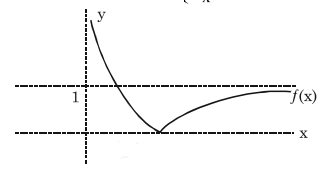Deepak Scored 45->99%ile with Bounce Back Crack Course. You can do it too!

# Let a function

Question:

Let a function $f:(0, \infty) \rightarrow(0, \infty)$ be defined by

$f(x)=\left|1-\frac{1}{x}\right| .$ Then $f$ is :-

1. Injective only

2. Not injective but it is surjective

3. Both injective as well as surjective

4. Neither injective nor surjective

Correct Option: , 2

Solution:

$f(x)=\left|1-\frac{1}{x}\right|=\frac{|x-1|}{x}=\left\{\begin{array}{cc}\frac{1-x}{x} & 0$\Rightarrow f(x)$is not injective but range of function is$[0, \infty)$Remark: If co-domain is$[0, \infty)$, then$f(x)\$ will be surjective